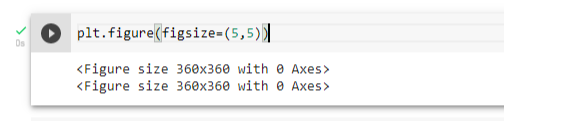top of pageSearch

# Intro to Matplotlib

Introduction :

Matplotlib is one of the most popular Python packages used for data visualization. It is a cross-platform library for making 2D plots from data in arrays. Matplotlib is written in Python and makes use of NumPy, the numerical mathematics extension of Python. It provides an object-oriented API that helps in embedding plots in applications using Python GUI toolkits such as PyQt, WxPythonotTkinter. Matplotlib along with NumPy can be considered as the open source equivalent of MATLAB.

Plotting :Let us import the python librariesFirst iam determining the size of the plot :

There are lot of functions to cover, let us start with linspace

linspace is an in-built function in Python's NumPy library. It is used to create an evenly spaced sequence in a specified interval.

Here let us assign NumPy (for Mathematics and working with arrays) to ‘X’ and add desired linestyle, markersize, linewidth etc., as shown below.Result :.

Line Plot :

In this line plot let us take the data of salary given to employees , their estimated salaries and the salary range in the market. To create the line plot between salary and est_sal let us use plot.plt(x,y).Result :Bar Chart :

In this bar chart let us use a new function add_axes() function. The add_axes() method figure module of matplotlib library is used to add an axes to the figure. This parameter is the dimensions [left, bottom, width, height] of the new axes. I want to plot a bar chart between Employee designation and their salaries according to their designation. The Axes. bar() function in axes module of matplotlib library is used to make a bar plot.Result :In the above code we used the Axes. bar() function in which axes module of matplotlib library is used to make a bar plot.

Pie Chart :

In this pie chart let us use add_axes() function. The add_axes() method figure module of matplotlib library is used to add an axes to the figure. This parameter is the dimensions [left, bottom, width, height] . On a pie chart what is not apparent is the axis, although it does have one. It is in fact the perimeter of the circle that serves as the axis. Autopct enables you to display the percent value using Python string formatting. For example, if autopct='%. 2f' , then for each pie wedge, the format string is '%. 2f' and the numerical percent value for that wedge is pct , so the wedge label is set to the string '%.Result :Scatter Plot :

In Scatter plot i have used scatter() Function. The Axes. scatter() function in axes module of matplotlib library is used to plot a scatter of y vs. x with varying marker size and/or color.Result :Conclusion :

We have seen how matplot library helps in visualizing and analyzing the data . It helps to understand large and complex amounts of data very easily.